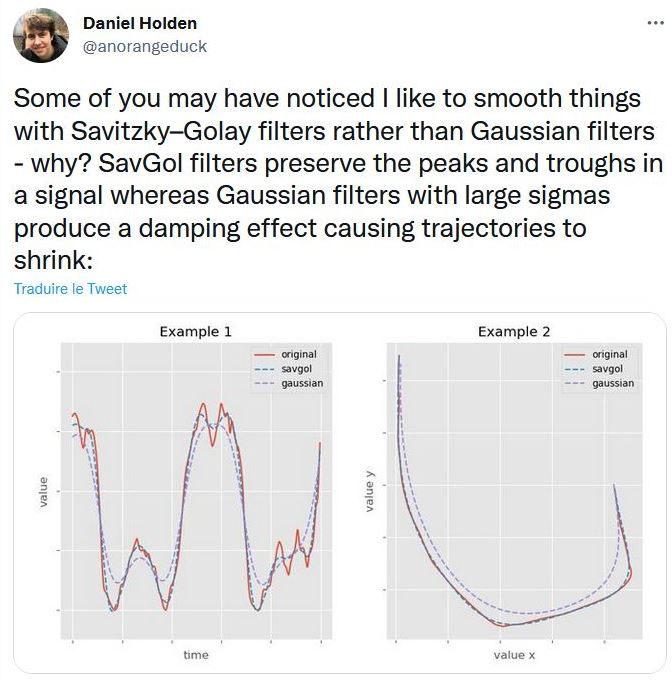# Simple animation smoothing algorithm

Consider the previous and next keyframe, do a linear interpolation to create an "intermediate" keyframe. Then do a linear interpolation between this newly created keyframe and the existing keyframe in the middle (or replace it altogether)

```t = (pose.secs - prevPose.secs) /
(nextPose.secs - prevPose.secs);

lerpPose = Lerp(prevPose, nextPose, t);
res= Lerp(pose, lerpPose, smoothStrength);
```

A more detailed version:

```
for (int j = 0; j < nb_smoothing_iterarions; ++j)
{

Animation<SkeletonPose> smoothed = new Animation<SkeletonPose>();
for (int i = 1; i < _cooked.keys.Count - 1; ++i)
{
SkeletonPose previousPose = _cooked.keys[i - 1];
SkeletonPose current = _cooked.keys[i];
SkeletonPose nextPose = _cooked.keys[i + 1];

float parameter = (current.seconds - previousPose.seconds) /
(nextPose.seconds - previousPose.seconds);

// Lerp every joint transformations inside the pose
// Works best when the skeleton uses quaternions:
SkeletonPose predictedPose = previousPose.Lerp(nextPose, parameter);
}

_cooked = smoothed;
}

```

Better smoothing algorithms exists such as Savitsky-Golay filters, however, they are way more involved.